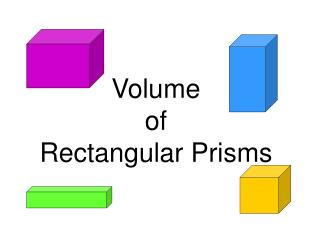DownloadDownload PresentationVolume of Rectangular Prisms

Volume of Rectangular Prisms

Télécharger la présentationVolume of Rectangular Prisms

- - - - - - - - - - - - - - - - - - - - - - - - - - - E N D - - - - - - - - - - - - - - - - - - - - - - - - - - -
Presentation Transcript

1. VolumeofRectangular Prisms

2. REVIEW - a three-dimensionalfigure encloses a part ofspace

3. Three-Dimensional Figures • faces – the flat surfaces • edges – the segments formed by intersecting faces • vertices – the points formed by intersecting edges

4. faces edges vertices

5. A three-dimensional figure encloses a part of space. • prism – has two parallel and congruent bases in the shape of polygons; the shape of the bases tells the name of the prism

6. A three-dimensional figure encloses a part of space. • pyramid – has a polygon for a base and triangles for sides; the shape of the base tells the name of the pyramid

7. A three-dimensional figure encloses a part of space. • cone – has curved surfaces, a circular base and one vertex

8. A three-dimensional figure encloses a part of space. • cylinder – has curved surfaces, two circular bases and no vertices

9. A three-dimensional figure encloses a part of space. • sphere – has no faces, bases, edges, or vertices; all the points are the same distance from a given point called the center

10. volume – the amount of space inside a three-dimensional figure The volume (V) of a rectangular prism equals the product of its length (l), its width (w), and its height (h). V = lwh h w l

11. volume – the amount of space inside a three-dimensional figure The volume (V) of a cube equals the product of three of its sides (s). V = s3 s s s

12. Find the volume of the rectangular prism. V = lwh V = 12 . 6 . 8 8m V = 72 . 8 6m V = 576 m3 12m

13. Find the volume of the rectangular prism. 20 ft V = lwh V = 20 . 5 . 6 6 ft 5 ft V = 100 . 6 V = 600 ft3

14. An Olympic-sized pool is 25 m wide, 50 m long, and 3 meters deep. What is the pool’s volume? V = lwh V = 50 . 25 . 3 V = 1250 . 3 V = 3750 m3